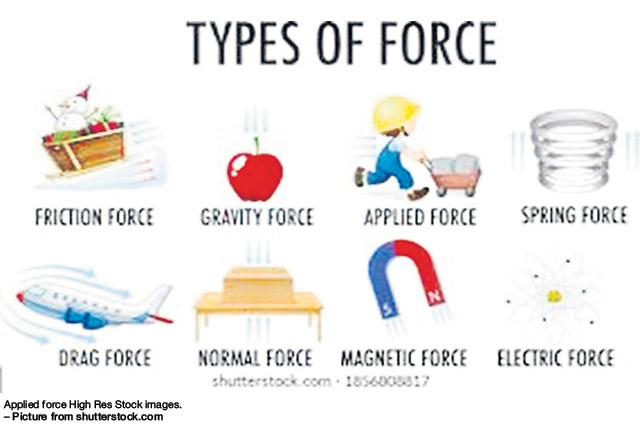# Work, power and energy

###### SCIENCE IN ACTIONBY MICHAEL JOHN UGLO
GOOD day one and all and welcome to our fourth lecture on work, power and energy in the Science in Action series.
Now prior to the lecture, I have announced my science, engineering and technology breakthrough product last week and asked you to pledge your support to be part of the team. We need a sponsor right now and it can be any individual, organisation or department, expect political affiliations.
You see, all of us cannot come first in a test in a classroom. It is going to be only one person who is going to top the class. Any invention, innovation and breakthrough is the same. All the nation’s struggle for science textbooks for biology, physics, geology and chemistry is solved in this breakthrough product. The icing on the cake is the technology, engineering, electronics and computer science that is built into this product to make it most appetising and revolutionising like never before. This is a patentable product that will not only solve PNG’s science, technology and engineering problems for the many generations to come but also this product will sell around the world to bring money into this country. How does that sound as an innovation?

Now to this week’s topic. Power, work and energy are all linked to one another. That is why in the first instances of their studies in physics are all put together. Power is the amount of work done per unit time and is measured in wattage. Work is a product of force applied in any direction with a displacement produced by that applied force. It is measured in a unit called joules. Then, combining all work done by a force as a sum results in the kinetic energy of the moving body or a moving particle. This points to the work-energy principle that contains this fact.
Power can be looked at in all applications as work done in unit time in any given instance by a moving substance or a particle in motion. In electricity, power is used in a similar manner considering current and the magnetic field. Power has components and is best looked at in its formula Power equals work over the time (P=W/t). Power is seen as a phenomenon derived out of time in work.

Working with the formula for work, it is the product of force and displacement. Hence, displacement being a vector quantity is multiplied with a particular size of force will give the magnitude for work.
Work is also measured in joules because it involves energy. Work is a scalar quantity measured in joules and energy is also a scalar quantity measured in joules as well. In combining work power and time, they can be given in the formula, is the transpose of the above formula as work equals work times time (W=Pt).
In mechanical power, it is the combination of forces and movements. The movements can be linear or rotational. Linear movements are basically the applied force multiplied by the displacement. The rotational movement involves a torque and the movement of the mechanical part like the shaft of a motor. Torque is the amount of force that causes an object to acquire rotation about an axis. The angular velocity of the shaft multiplied by the torque in degrees of radians will give the size of the mechanical power.

To the point where experiences no power losses, the input power must equal to the output power. There is a 100 per cent efficiency of the power output from the initial power input. This is called a mechanical advantage and is given in the formula P = FBvB = FAvA. Therefore, the mechanical advantage of the output power resulting from the input power is given as
MA = FB/FA = vA/vB
In the case of the systems that rotate, there is a very similar relation to the linear system as given above. The Ta and wA are the torque and the angular velocity respectively. Rotating systems have the arrangement written as P = TAwA = TBwB. The mechanical advantage formula is MA = TB/TA = wA/wB. The symbol MA stands for the mechanical advantage.
In the case of the electrical power, instantaneous power discharged for a system is basically the current multiplied by the voltage as according to the ohms law as voltage equals current times the resistance. The similarity is that all these quantities are given as a derivative of time because it is all instantaneous. Thus, the formula is P = I x V and can be all considered as a derivative of time as P(t) = I(t) x V(t). If a resistance is considered in this arrangement without a change in time then, the formula for power will be power equals to current squared times the resistance given as P = I2 x R. This will equal to V2/R whereby the resistance will equal to voltage over the current written as R = V/I. This in essence is the formula for the ohms law which is voltage equals to Current times the Resistance. That is V = IR. Power from an initial and final stages whether in linear or rotational can be calculated with the use of mathematical calculus in derivative and integration. They can be derived as a function of time for instantaneous power output. Such formula as Wc = ∫c F.vdt = ∫c F.dx where F is the force and c is the circular path the body or particle is taking while v is the velocity along this path.
A mass can travel with a particular magnitude of acceleration as exerted by the force.